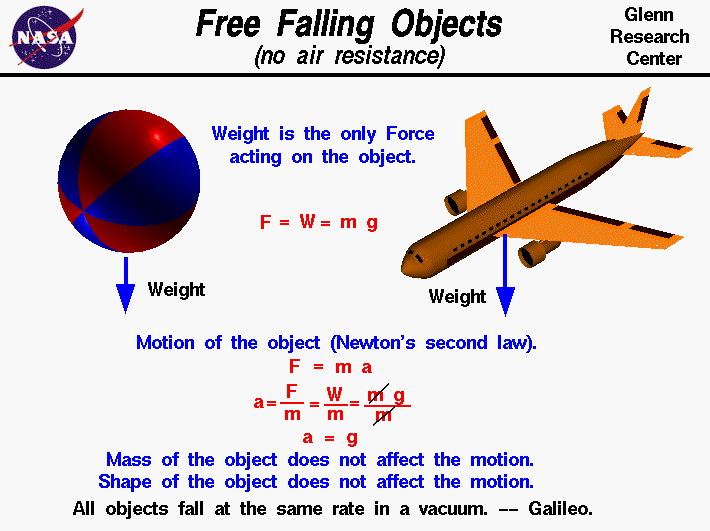+ Text Only Site
+ Non-Flash Version
+ Contact GlennAn object that falls through a vacuum is subjected to only one external force, the gravitational force, expressed as the weight of the object. The weight equation defines the weight W to be equal to the mass of the object m times the gravitational acceleration g: W = m * g the value of g is 9.8 meters per square second on the surface of the earth. The gravitational acceleration g decreases with the square of the distance from the center of the earth. But for many practical problems, we can assume this factor to be a constant. An object that moves because of the action of gravity alone is said to be free falling. If the object falls through the atmosphere, there is an additional drag force acting on the object and the physics involved with the motion of the object is more complex. The motion of any moving object is described by Newton's second law of motion, force F equals mass m times acceleration a: F = m * a We can do a little algebra and solve for the acceleration of the object in terms of the net external force and the mass of the object: a = F / m For a free falling object, the net external force is just the weight of the object: F = W Substituting into the second law equation gives: a = W / m = (m * g) / m = g The acceleration of the object equals the gravitational acceleration. The mass, size, and shape of the object are not a factor in describing the motion of the object. So all objects, regardless of size or shape or weight, free fall with the same acceleration. In a vacuum, a beach ball falls at the same rate as an airliner. Knowing the acceleration, we can determine the velocity and location of any free falling object at any time. The remarkable observation that all free falling objects fall with the same acceleration was first proposed by Galileo Galilei nearly 400 years ago. Galileo conducted experiments using a ball on an inclined plane to determine the relationship between the time and distance traveled. He found that the distance depended on the square of the time and that the velocity increased as the ball moved down the incline. The relationship was the same regardless of the mass of the ball used in the experiment. The experiment was successful because he was using a ball for the falling object and the friction between the ball and the plane was much smaller than the gravitational force. He also used a very shallow incline, so the velocity was small and the drag on the ball was very small compared to the gravitational force. (The story that Galileo demonstrated his findings by dropping two cannon balls off the Leaning Tower of Pisa is just a legend.). Activities: Guided ToursFalling Objects:Navigation ..Beginner's Guide Home Page+ Inspector General Hotline + Equal Employment Opportunity Data Posted Pursuant to the No Fear Act + Budgets, Strategic Plans and Accountability Reports + Freedom of Information Act + The President's Management Agenda + NASA Privacy Statement, Disclaimer, and Accessibility CertificationEditor: Nancy Hall NASA Official: Nancy Hall Last Updated: May 13 2021 + Contact Glenn